Smartick is a fun way to learn math!# I want to learn about: Decimals

Learn about decimal numbers with Smartick. Exercises, tutorials problems, and teaching resources about decimal numbers for elementary aged children. Here you will find posts about decimal numbers to make learning mathematics easier and more fun.

Nov11

## Multiplication of Decimals Using Money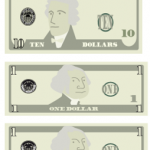Today we are going to better understand the multiplication of decimals by using a very common manipulative material: money. It is going to help us understand why multiplying by 10 moves the decimal position to the right. Equivalence of Bills or Coins with Numbers First, we are going to establish the equivalence of each coin […]

Aug05

## Adding and Subtracting Decimal Numbers Using Money as an Example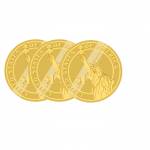To put the addition and subtraction of decimal numbers into practice with money, it is essential to have previous knowledge about decimals and their representation with money. Once we have mastered this knowledge, it’s time to start solving problems. Steps to add and subtract decimal numbers: Place the numbers in columns in such a way […]

Jul08

## Dividing with Decimals Using Money as an Example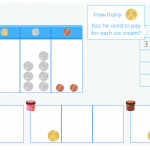In today’s post, you are going to learn how to solve division problems with decimals in the dividend, in other words, dividing a decimal number by a whole number. In order for you to understand the process well, I am going to show you how we explain dividing with decimals in the interactive Smartick tutorial […]

May06

## Using Money to Help Learn Decimal Numbers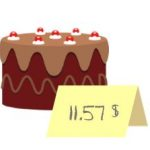Today’s post deals with the day to day use of decimal numbers using money as an example (and to help you better understand it). We are going to start with the basics, reviewing what decimal numbers are and how to read and write them. You can use these resources to refresh your memory: What are […]

Feb17

## Combining Decimals and Fractions

Imagine that you have to solve the following addition problem that combines decimals and fractions: It’s not so easy to add a fraction to a decimal, right? It’s much easier to add fractions to fractions, or decimals to decimals. So, we can do this in two ways: Change the decimal to a fraction. Change the […]

Jan27

## Learn How to Do Division Word Problems with DecimalsToday we are going to review division word problems with decimals. Does it seem difficult? Well, it’s not! Solving division word problems with decimals is the same as solving them with whole numbers. I’m going to show you! You can review what a decimal number is here. Every time we solve a word problem, the first […]

Jan06

## How to Round to the Nearest Whole Number. Estimations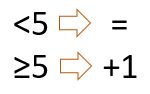Rounding decimals to the nearest whole number or the tenths, hundredths, thousandths is easy! In this post, we are going to learn to round decimal numbers. Rounding decimals helps us to manage the numbers more easily in order to operate them. Rounding decimals to the nearest whole number To round a number to the nearest whole […]